GCSE Maths Number

Factors, Multiples And Primes

# Factors, Multiples And Primes

Here we will learn about factors and multiples and primes, including what they are and how they can be used.

There are also factors, multiples and primes worksheets based on Edexcel, AQA and OCR exam questions, along with further guidance on where to go next if you’re still stuck.

## What are factors, multiples and primes?

Factors, multiples and primes are different types of numbers.

• A factor is a number which divides into another number exactly with no remainders.

For example,

What are the factors of 12 ?

To do this we can think of pairs of numbers which multiply together to make 12 .

\begin{aligned} &1\times 12 \\\\ &2\times 6 \\\\ &3\times 4 \end{aligned}

So the factors of 12 are: 1, 2, 3, 4, 6 and 12.

Step-by-step guide: Factors

• A multiple of a number is a number in its times table.

For example,

The multiples of 4 are: 4, 8, 12, 16, 20 and so on.

Step-by-step guide: Multiples

• A prime number is a number that only has two factors, 1 and itself.

The first few prime numbers are: 2, 3, 5, 7, 11, 13, 17, 19, 23 and so on.

Step-by-step guide: Prime numbers

### What are factors, multiples and primes?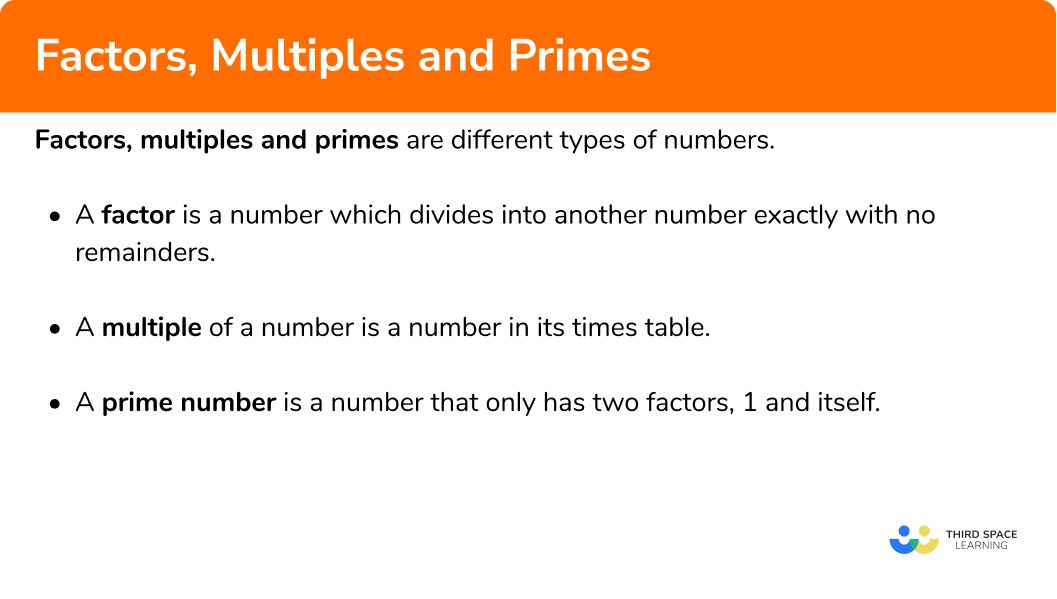## How to use factors, multiples and primes

We can use factors, multiples and primes to work with:

• Prime factors
• Factor trees
• Lowest common multiple (LCM)
• Highest common factor (HCF)

### How to use factors, multiples and primes?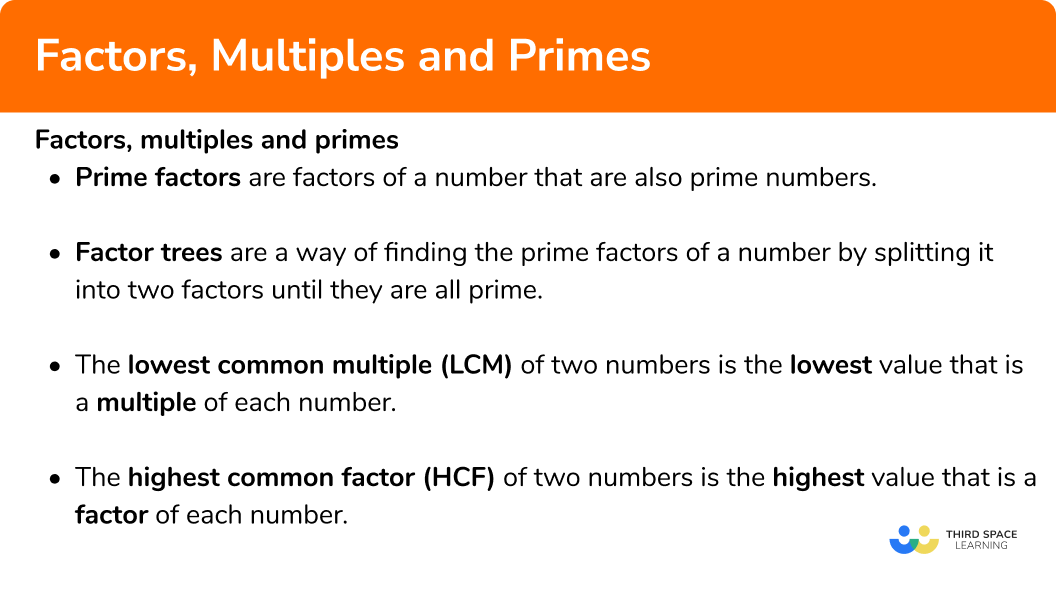### Prime factors

Prime factors are factors of a number that are also prime numbers. We can write any number as a product of its prime factors – this is called prime factorisation (prime factorization).

For example,

A composite number is a non-prime number which is the product of two or more prime factors. All of these types of numbers are integers (whole numbers).

Step-by-step guide: Prime factors

### Factor trees

• Factor trees are a way of finding the prime factors of a number.  This is useful when asked to write a number as a product of its prime factors.

For example,

A factor tree for 150 may look like this,

Each branch in the tree is split into two factors which multiply together to make the number they are branching from. Once the factor at the end of the branch is a prime number, its only two factors are itself and one so the branch stops and we circle the number.

Here is 150 as a product of its prime factors,

150=3\times 5\times 2\times 5=2\times 3\times 5^2 .

Step-to-step guide: Factor trees

### Lowest common multiple

• The lowest common multiple (LCM) or least common multiple is the smallest integer that belongs to the multiplication table of two or more numbers – it is the lowest value that is a multiple of each number.

For example,

Find the LCM of 4 and 5 .

Let’s start by writing the multiples of 4 and 5 .

Multiples of 4: \quad \quad 4, 8, 12, 16, 20, 24, 28, 32, 36, 40, 44, …

Multiples of 5: \quad \quad 5, 10, 15, 20, 25, 30, 35, 40, 45, ...

We can see that the smallest positive integer that occurs in each list is 20 , so the lowest common multiple of 4 and 5 is \bf{20.}

Note: 40 occurs in both lists so is a common multiple, but not the lowest common multiple.

Step-to-step guide: Lowest common multiples

### Highest common factor

• The highest common factor (HCF) or greatest common factor is the largest integer that two or more numbers can be divided by – it is the highest value that is a factor of each number.

For example,

Find the HCF of 12 and 20 .

Let’s start by writing the factors of 12 and 20 .

We can see the highest number that occurs in each list is 4, hence the highest common factor of 12 and 20 is 4.

Note: 1 and 2 occur in both lists, so they are common factors, but not the highest common factor.

Step-to-step guide: Highest common factor

## Factors and multiples examples

### Example 1: listing factors

List the factors of 18 .

Use factor pairs, starting from 1, then 2 and so on.

\begin{aligned} 18&= 1\times 18\\\\ &=2\times 9\\\\ &=3\times 6 \end{aligned}

The factors of 18 are: 1, 2, 3, 6, 9 and 18 .

### Example 2: listing multiples

Write down the first 6 multiples of 7 .

\begin{aligned} &1\times 7=7 \\\\ &2\times 7=14 \\\\ &3\times 7=21 \\\\ &4\times 7=28 \\\\ &5\times 7=35 \\\\ &6\times 7=42 \end{aligned}

The first 6 multiples of 7 are: 7, 14, 21, 28, 35 and 42 .

### Example 3: prime factors

Write 42 as a product of its prime factors.

One way is to divide the number by prime numbers and continue to do so until the final answer is a prime number.

First divide by 2 ,

42\div 2 = 21 .

Then divide by 3 ,

21\div 3=7 .

7 is prime so we can stop.

So,

42=2\times 3\times 7

\begin{aligned} 42&= 2\times 21\\\\ &=2\times 3\times 7 \end{aligned}

42 as a product of its prime factors is 2 \times 3 \times 7 .

### Example 4: factor trees

Construct a factor tree for 180 .

180=18\times 10

Then continue to construct branches from the 18 and the 10. Continue until each branch ends with a prime number.

We can write 180 as a product of its prime factors,

180=2\times 3\times 3\times 2\times 5 .

Or in numerical order,

180=2\times 2\times 3\times 3\times 5 .

Or in index form,

180=2^2\times 3^2\times 5 .

Note: Most numbers have many possible factor trees.

### Example 5: lowest common multiple

Find the lowest common multiple of 12 and 20 .

Multiples of 12 are: \quad 12, 24, 36, 48, 60, 72 …

Multiples of 20 are: \quad 20, 40, 60, 80 …

The LCM of 12 and 20 is 60.

Alternatively you can use prime factorisation and Venn diagrams.

Write the numbers as products of their prime factors.

\begin{aligned} &12=2\times 2\times 3 \\\\ &20=2\times 2\times 5 \end{aligned}

Put the prime factors into a Venn diagram.

Multiply each prime factor in the Venn diagram to find the LCM.

3\times 2\times 2\times 5=60

The LCM of 12 and 20 is 60.

### Example 6: highest common factor

Find the highest common factor of 30 and 48 .

\begin{aligned} 30&= 1\times 30\\\\ &=2\times 15\\\\ &=3\times 10\\\\ &=5\times 6 \end{aligned}

\begin{aligned} 48&= 1\times 48\\\\ &=2\times 24\\\\ &=3\times 16\\\\ &=4\times 12\\\\ &=6\times 8 \end{aligned}

The factors of 30 are: \quad \quad 1, 2, 3, 5, 6, 10, 15 and 30

The factors of 48 are: \quad \quad 1, 2, 3, 4, 6, 8, 12, 16, 24 and 48

The HCF of 30 and 48 is 6.

Alternatively you can use prime factorisation and Venn diagrams.

Write the numbers as products of their prime factors.

30=2\times 3\times 5

48=2\times 2\times 2\times 2\times 3

Put the prime factors into a Venn diagram.

Multiply the prime factors in the intersection to find the HCF.

2\times 3=6

The HCF of 30 and 48 is 6.

### Common misconceptions

• 1 and prime numbers

1 is not a prime number. This is because it only has one factor, rather than the 2 factors needed to be a prime number.

• Factors and multiples

Factors and multiples are easily mixed up. Remember multiples are the multiplication table, whereas factors are the numbers that go into another number without a remainder.

• Remember the number itself for factors and multiples

All numbers are a factor of themselves and a multiple of themselves.

For example,

Think of the number 6.

The factors of 6 are: 1, 2, 3, 6.

The multiples of 6 are: 6, 12, 18, 24 and so on.

So 6 is a factor and a multiple of itself.

### Practice factors and multiples questions

1. List the factors of 50 .

50, 100, 150, 200, 250 …1, 2, 5, 10, 25, and 502, 5, 10 and 252\times 5^2To list the factors of 50 we can use factor pairs.

\begin{aligned} 50&= 1\times 50\\\\ &=2\times 25\\\\ &=5\times 10 \end{aligned}

So the factors of 50 are 1, 2, 5, 10, 25 and 50.

2. List the first 4 multiples of 15 .

1, 3, 5 and 151+2+3+4+53\times 515, 30, 45 and 60We need the first 4 numbers of the 15 times table.

\begin{aligned} &1\times 15=15\\\\ &2\times 15=30\\\\ &3\times 15=45\\\\ &4\times 15=60 \end{aligned}

So, the first 4 multiples of 15 are: 15, 30, 45 and 60.

3. Write 120 as a product of its prime factors.

2,2,2,3,52^3 \times 3\times 52\times 3\times 512\times 10Divide 120 by 2 and other primes until you are left with a prime number.

\begin{aligned} 120&= 2\times 60\\\\ &=2\times 2\times 30\\\\ &=2\times 2\times 2\times 15\\\\ &=2\times 2\times 2\times 3\times 5 \end{aligned}

We can then write the product of prime factors in index form,

120=2^3 \times 3\times 5 .

4. Which is an incorrect factor tree for 144 ?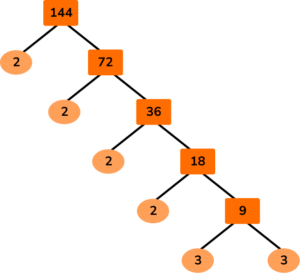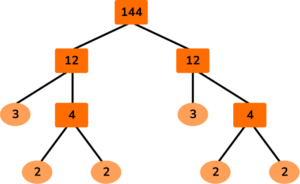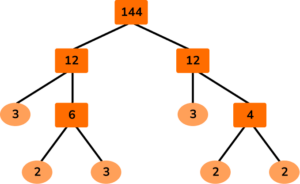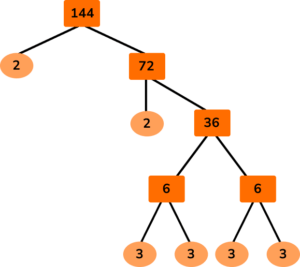The branches from a number need to multiply together to make that number.

3 \times 3 6

Also, the prime numbers should all multiply together to make 144.

For option D,

2\times 2\times 3\times 3\times 3\times 3=324 .

5. Find the lowest common multiple of 8 and 10.

2024080Write down the multiples of 8 and the multiples of 10 and see which is the lowest number that occurs in both lists.

Multiples of 8: \quad 8, 16, 24, 32, 40, 48, …

Multiples of 10: \;\; 10, 20, 30, 40, 50, …

So, 40 is the LCM of 8 and 10.

Alternatively you can use prime factorisation and Venn diagrams.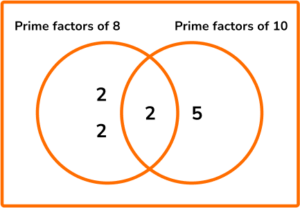\text{LCM}=2\times 2\times 2\times 5=40

6. Find the highest common factor of 56 and 70 .

7282114List the factors of 56 and 50 and see which is the highest number in both lists.

Factors of 56 are: \quad 1, 2, 4, 7, 8, 14, 28 and 56

Factors of 70 are: \quad 1, 2, 7, 10, 14, 35 and 70

So, 14 is the HCF of 56 and 70 .

Alternatively you can use prime factorisation and Venn diagrams.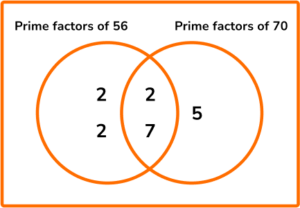The HCF is the product of the prime factors in the intersection.

\text{HCF}=2\times 7=14

### Factors and multiples GCSE questions

1. (a) Write down a multiple of 7 between 20 and 30 .

(b) Write down two factors of 70 that are prime numbers.

(3 marks)

(a)

21 or 28

(1)

(b)
One answer from 2, 5, 7 .

(1)

Another one from 2, 5, 7 .

(1)

2. (a) Find the highest common factor of 22 and 77 .

(b) Find the lowest common multiple of 22 and 77 .

(4 marks)

(a)

Factors of 22: 1, 2, 11 and 22

Factors of 77: 1, 7, 11, and 77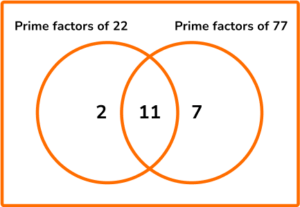(1)

HCF = 11

(1)

(b)

Multiples of 22: 22, 44, 88, 110, 132, 154, …

Multiples of 77 are: 77, 154, 231, …

\text{LCM}=2\times11\times7

(1)

LCM = 154

(1)

3. Two buses leave the depot at 9 \; am.

Bus B takes 55 minutes to complete its journey and return to the depot.

Bus B takes 55 minutes to complete its journey and return to the depot.

What time is it when both buses are back at the depot together?

(4 marks)

\begin{aligned} &45=3\times 3\times 5 \\\\ &55=5\times 11 \end{aligned}

(1)

\text{LCM}=3\times3\times5\times11=495

(1)

495=8.25 \ \text{hours}

(1)

17:15 or 5:15 \ \text{pm}

(1)

## Learning checklist

You have now learned how to:

• Find factors and multiples
• Write numbers as a product of their prime factors
• Find the lowest common multiple
• Find the highest common factor

## Still stuck?

Prepare your KS4 students for maths GCSEs success with Third Space Learning. Weekly online one to one GCSE maths revision lessons delivered by expert maths tutors.

Find out more about our GCSE maths tuition programme.